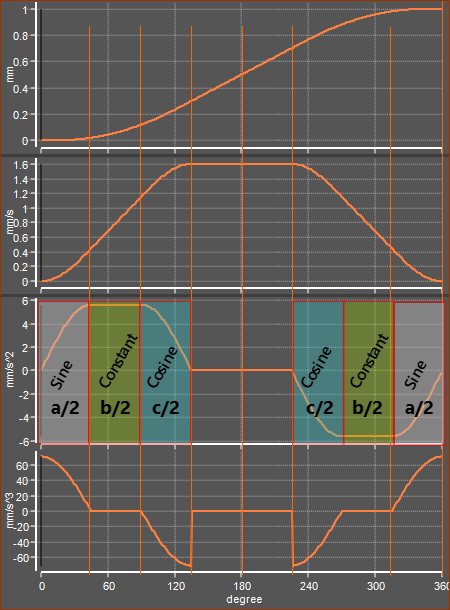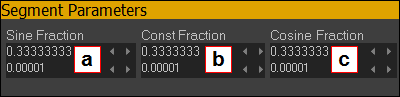﻿ MotionDesigner Reference & User Interface > Motion Laws > Sine-Constant-Cosine (SCCA) Motion-Law

# Sine-Constant-Cosine (SCCA) Motion-Law

Navigation:  MotionDesigner Reference & User Interface > Motion Laws >

# Sine-Constant-Cosine (SCCA) Motion-Law

### Sine-Constant-Cosine Acceleration (SCCA) Cam-Law, Motion-Law

Sine-Constant-Cosine Acceleration

Segment-Parameters

The Segment-Parameters make this motion-law very flexible. It is possible to get many of the Traditional Motion-Laws that are based on Sine and Cosine harmonics.

You CAN specify the:

Start-Position (usually flows from the End-Position of the Previous-Segment )

End-Position

You CANNOT specify the:

Start-Velocity, End-Velocity - Zero

Start-Acceleration, End-Acceleration - Zero

Start-Jerk, End-Jerk - Finite

The SCCA motion-law is a defined by a sequence of trigonometric segments in acceleration motion-derivative. The motion-law is symmetrical, thus the sequence is:

0.0 ≤ X ≤ 0.5

a.Sine Fraction

b.Constant Fraction

c.Cosine Fraction:

d.Zero Acceleration

0.5 ≤ X ≤ 1

a.Zero Acceleration

b.Cosine Fraction

c.Constant Fraction

d.Sine Fraction

Segment Parameters

a = Total Sine Acceleration Fraction (the acceleration at the start + deceleration at the end)

b = Total Constant Acceleration Fraction

c = Total Cosine Acceleration Fraction

a + b + c + d = 1

Enter only a, b, & c.

d = 1 – (a + b + c)

d = Total Zero Acceleration Fraction

Rules for the segment parameters:

Segment-Parameters may be zero, but not all.

No Segment-Parameter can be negative.

An Example Sine Constant-Cosine Acceleration Law.Example motion with Sine-Constant-Cosine Motion Law with Segment Parameters: Sine Fraction a=0.333, Constant b=0.333, Cosine Fraction c=0.333Segment Parameters in the example Motion-Law - see images to left a = 0.333 ; 33.3% : Sine Acceleration Fraction b = 0.333 ; 33.3% : Constant Acceleration Fraction c = 0.333 ; 33.3% : Cosine Acceleration Fraction ∴ d = 1 – a – b – c = 0.0 ; 0% : of the Zero Acceleration Fraction.

### Sine-Constant-Cosine Segment Parameters to give other Traditional Motion-Laws.

MOTION-LAW:

Coefficients

Parameters

Cv

Ca

a

b

c

Modified Trapezoidal:

2

4.89

0.25

0.5

0.25

'Lazy' Modified Trapezoidal:

2

4.88

0.333

0.333

0.333

Modified-Sine:

1.760

5.5

0.25

0

0.75

Modified-Sine CV 20%:

1.528

5.999

0.2

0

0.6

Modified-Sine CV 33%:

1.404

6.616

0.1667

0

0.5

Modified-Sine CV 50%:

1.275

8.0127

0.125

0

0.375

Modified-Sine CV 66%:

1.168

11.009

0.0833

0

0.25

Cycloidal:

2

6.2832

0.5

0

0.5

Cycloidal CV 50%:

1.333

8.378

0.25

0

0.25

Constant Acceleration-Deceleration:

2

4

0

1

0

Trapezoidal Velocity CV33%:

1.5

4.5

0

0.6667

0